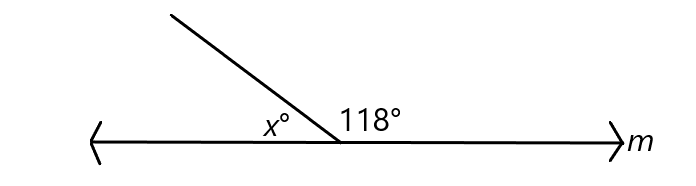﻿ Supplementary angles and examples - MathBootCamps

# Supplementary angles and examples

Supplementary angles are angles whose measures sum to 180°. In the lesson below, we will review this idea along with taking a look at some example problems.

In the image below, you see one of the common ways in which supplementary angles come up. The angles with measures $$a$$° and $$b$$° lie along a straight line. Since straight angles have measures of 180°, the angles are supplementary.## Example problems with supplementary angles

Let’s look at a few examples of how you would work with the concept of supplementary angles.

### Example

In the figure, the angles lie along line $$m$$. What is the value of $$x$$?### Solution

The two angles lie along a straight line, so they are supplementary. Therefore: $$x + 118 = 180$$. Solving this equation:
\begin{align}x + 118 &= 180\\ x &= \boxed{62} \end{align}

### Example

The angles $$A$$ and $$B$$ are supplementary. If $$m\angle A = (2x)^{\circ}$$ and $$m\angle B = (2x-2)^{\circ}$$, what is the value of $$x$$?

### Solution

Since the angles are supplementary, their measures add to 180°. In other words: $$2x + (2x – 2) = 180$$. Solving this equation gives the value of $$x$$.

\begin{align}2x + (2x – 2) &= 180\\ 4x – 2 &= 180\\ 4x &= 182\\ x &= \boxed{45.5} \end{align}

The previous example could have asked for some different information. Let’s look at a similar example that asks a slightly different question.

### Example

The angles $$A$$ and $$B$$ are supplementary. If $$m\angle A = (2x+5)^{\circ}$$ and $$m\angle B = (x-20)^{\circ}$$, what is $$m \angle A$$?

### Solution

This time you are being asked for the measure of the angle and not just $$x$$. But, the value of $$x$$ is needed to find the measure of the angle. So, first set up an equation and find $$x$$.

\begin{align}2x+5 + x – 20 &= 180\\ 3x-15 &= 180 \\ 3x &= 195\\ x&= 65\end{align}

The measure of angle $$A$$ is then:
$$m\angle A = (2x+5)^{\circ}$$ and $$x = 65$$

$$m\angle A = (2(65)+5)^{\circ} = \boxed{135^{\circ}}$$

## Summary

There isn’t much to working with supplementary angles. You just have to remember that their sum is 180° and that any set of angles lying along a straight line will also be supplementary.## Subscribe to our Newsletter!

We are always posting new free lessons and adding more study guides, calculator guides, and problem packs.

Sign up to get occasional emails (once every couple or three weeks) letting you know what's new!